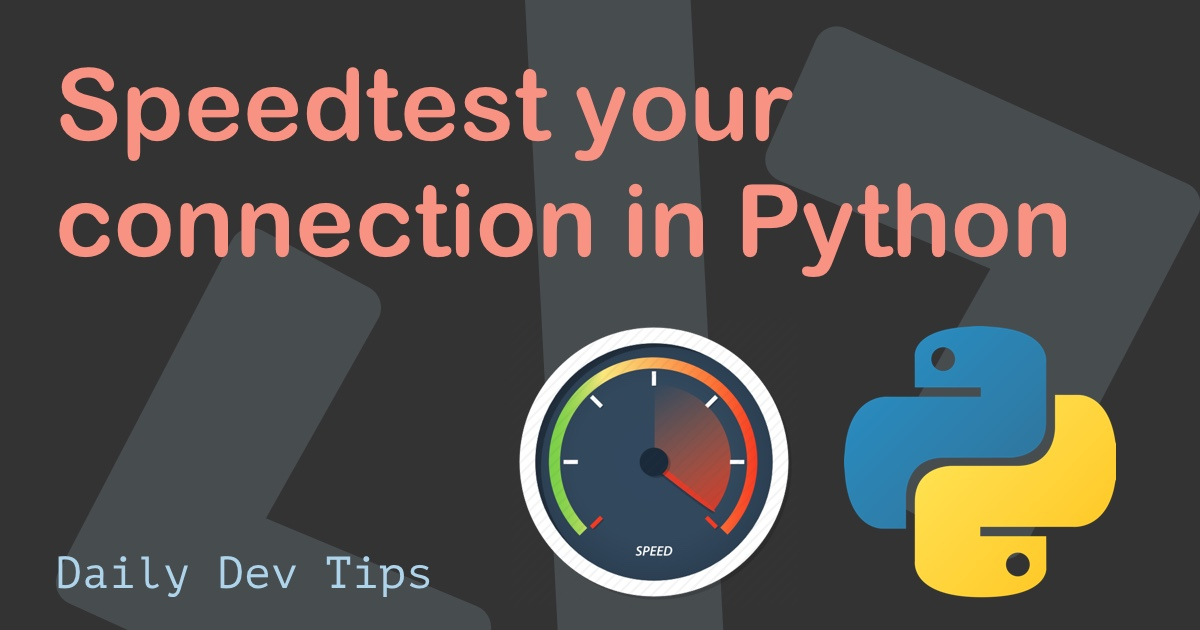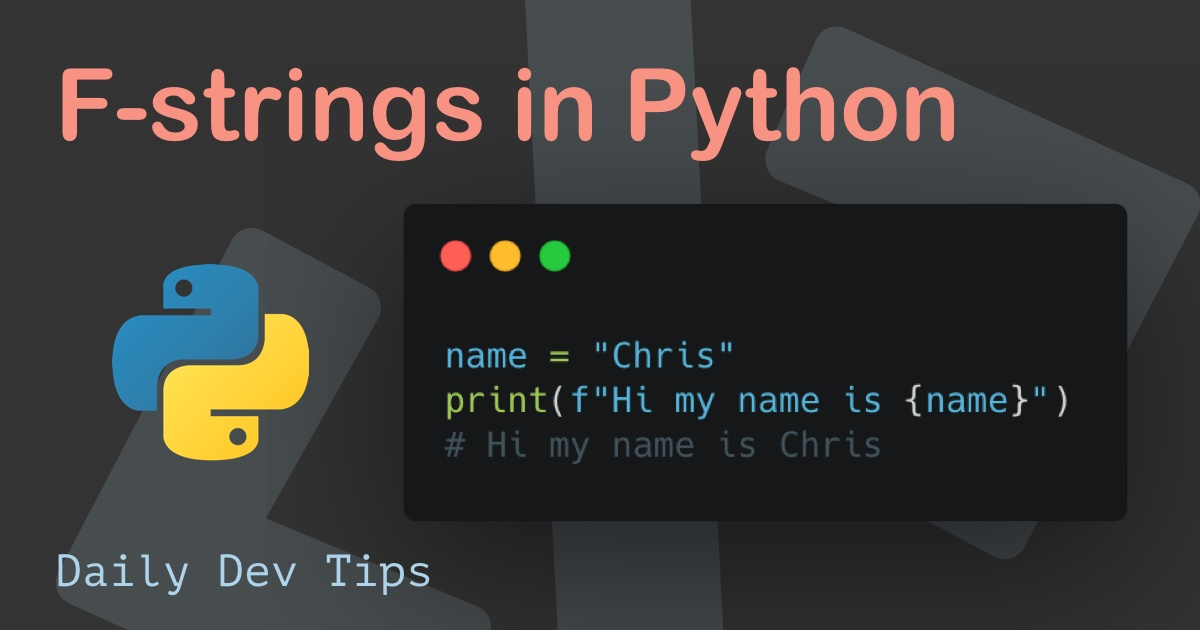Subscribe

# Installing and using NumPy in Python

••••••• + 4 devs liked it

✍️

What is NumPy and how to use it in Python

2 Jun, 2021 · 2 min read

First of all, let me explain a bit what NumPy is and why you might need it. NumPy is a Python library that is used for working with arrays. It stands short for Numeric Python

This, of course, is still a bit vague. In general, it makes working with arrays (lists) about 50x faster than traditional python lists.

## Installing and using NumPy permalink

To install NumPy, we must run a pip install command for it.

``pip install numpy``

Then we have to import it into our Python file.

``import numpy``

Now we can convert a list into a numpy array:

``````arr = numpy.array([1, 2, 3, 4, 5])
print(arr)``````

However, it's quite often used to have the numpy imported as the `np` alias.

We can do so like this:

``````import numpy as np

arr = np.array([1, 2, 3, 4, 5])
print(arr)``````

This now does the exact same thing, but it's easier to write.

If you ever wonder what version of numpy you have installed, you can simply print that out.

``````print(np.__version__)
# 1.20.3``````

The cool part about the NumPy arrays is that they can be built from all array-like data types of Python.

Which include the list, tuple, dictionary.

``````tuple = np.array((1, 2, 3, 4, 5))
print(tuple)

list = np.array(["dog", "cat", "penguin"])
print(list)

set = np.array({"dog", "cat", "penguin"})
print(set)``````

It's super easy to convert this stuff to NumPy arrays since we can eventually do more stuff and faster!

In a follow-up article, I'll go more in-depth about the options for the NumPy arrays.

Tweet this tip
••••••• + 4 devs liked it

### Speedtest your connection in Python

21 Jun, 2021 · 2 min read### F-strings in Python

7 Jun, 2021 · 2 min readJoin 2101 devs and subscribe to my newsletter

• 1000 articles written
• 2101 devs subscribed
• 529116 words written